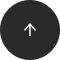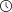- 4 minutes read

# SQL JOINs

A SQL JOIN is a method to retrieve data from two or more database tables. This article presents a basic overview of what data from a particular SQL join will look like. A popular way of understanding SQL joins is to visualize them using Venn diagrams, so each example have corresponding Venn diagram, appropriate `SELECT` statement and the result table.

There are a few major kinds of SQL joins:

• INNER JOIN
• OUTER [LEFT | RIGHT | FULL] JOIN
• NATURAL JOIN
• CROSS JOIN

We distinguish the implementation of these joins based on the join operators:

• equi and
• theta, which will be described later.For the purposes of this article, let's discuss joins using a simple example. Assume that we have two basic tables, TableA and TableB, which are filled with some example data. Since we'll be joining tables on `name` column, we distinguish the rows of the same name by highlighting them red.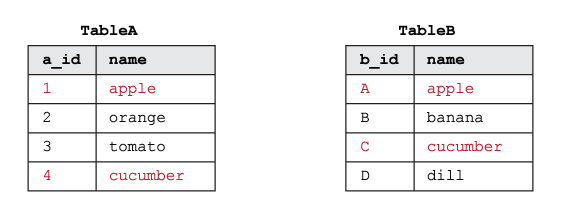In the following sections, we'll look at what happens to this data when different types of joins are implemented.

### CROSS JOIN

A CROSS JOIN is a Cartesian product of TableA and TableB. Every row from TableA is matched with every row from TableB; that's why a CROSS JOIN doesn't make sense in most situations.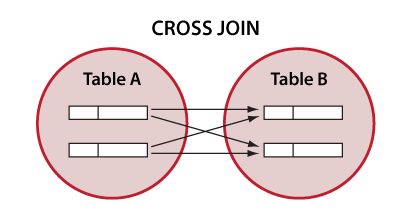```SELECT *
FROM tableA
CROSS JOIN tableB;
```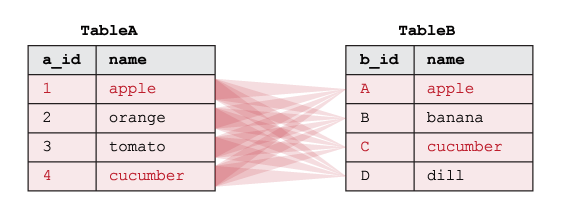TableA and TableB contain 4 rows. The resulting table will have 4 * 4 = 16 rows and will look as follows: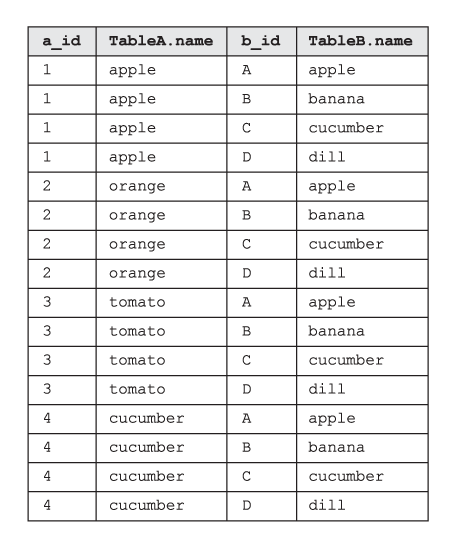### INNER JOIN

An INNER JOIN merges ONLY the matching rows in BOTH tables. A JOIN without any other JOIN keywords (like INNER, OUTER, LEFT, etc) is an INNER JOIN. Results are found in the overlapping area.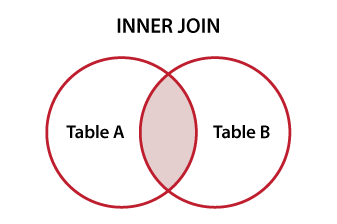```
SELECT *
FROM tableA
INNER JOIN tableB
ON tableA.name = tableB.name
```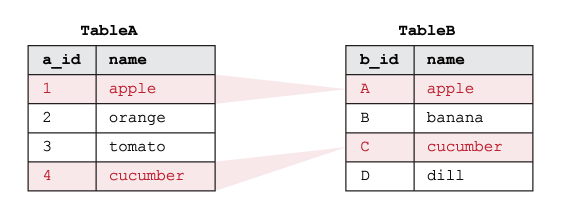The resulting table will be as follows: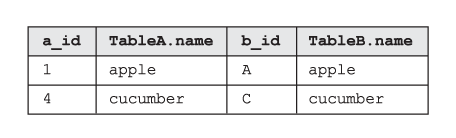### OUTER JOINS

FULL OUTER JOIN returns matched and unmatched rows from both tables (it's an union of both). If there is no match, the missing side will contain null.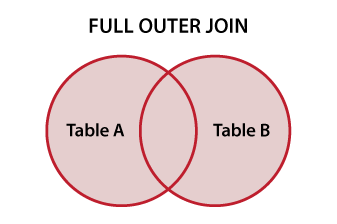```
SELECT *
FROM TableA
FULL OUTER JOIN TableB
ON TableA.name = TableB.name;```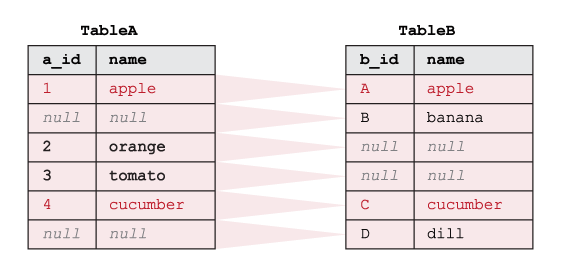The resulting table will be as follows: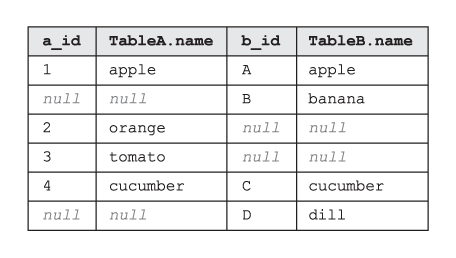A LEFT OUTER JOIN returns all rows from the left table (TableA) with the matching rows from the right table (TableB) or null – if there is no match in the right table.

The results can be found in the entire left circle: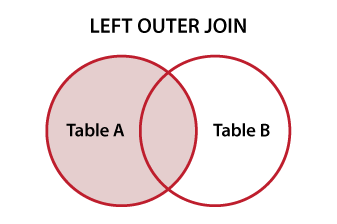```SELECT *
FROM TableA
LEFT OUTER JOIN TableB
ON tableA.name = tableB.name;
```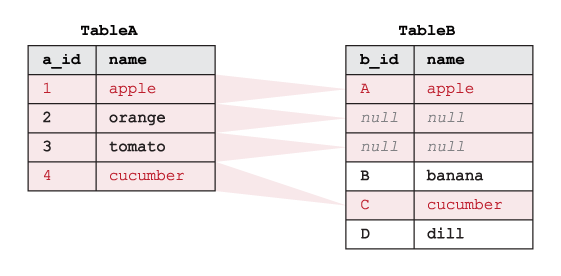The resulting table will be as follows: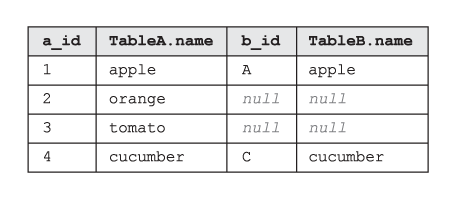A RIGHT OUTER JOIN returns all rows from the right table (TableB) with the matching rows from the left table (TableA) or null – if there is no match in the left table.

The results can be found in the entire right circle: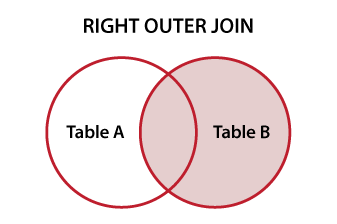```SELECT *
FROM tableA
RIGHT OUTER JOIN tableB
ON tableA.name = tableB.name```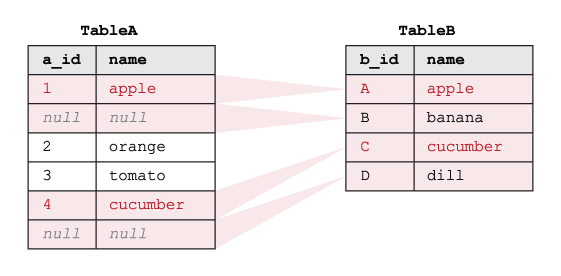The resulting table will be as follows: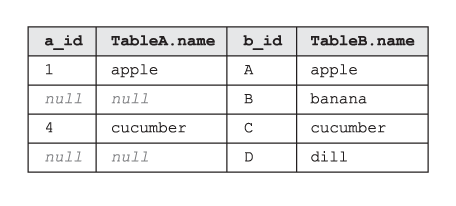### Joins based on operators

Equi-join implementation

This JOIN is made by using the equality-operator (=) to compare values of the PrimaryKey of one table and the Foreign Key values of another table.

```SELECT *
FROM TableA
INNER/OUTER JOIN TableB
ON TableA.PK =TableB.Fk;
```

Theta-join implementation (non-equi)

This is the same as the equi JOIN but it allows all other operators like >, &lt, >= etc.

```SELECT *
FROM TableA
INNER/OUTER JOIN TableB
ON tableA.Pk <= tableB.Fk;
```

Self-join implementation

This type of JOIN is usually used in case of a unary relationship type, where a table is combined with itself.

```SELECT *
FROM TableA A1
JOIN TableA A2
ON A1.Pk = A2.Fk;
```

### NATURAL JOIN

A NATURAL join is a type of EQUI join. There is no need to use an ON clause. Columns with the same name in associated tables appear once only.

```SELECT *
FROM tableA
NATURAL JOIN tableB
```

By manipulating keywords we can exclude specific data.

An OUTER EXCLUDING JOIN returns all of the records in TableA and all of the records in TableB that don't match.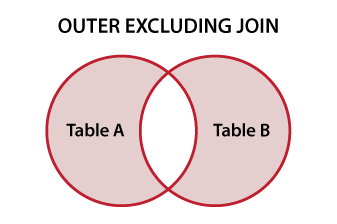```SELECT *
FROM tableA
FULL OUTER JOIN tableB
ON tableA.name = tableB.name
WHERE tableA.name IS NULL
OR tableB.name IS NULL
```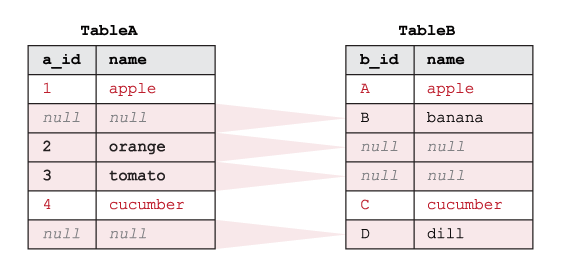The resulting table will be as follows: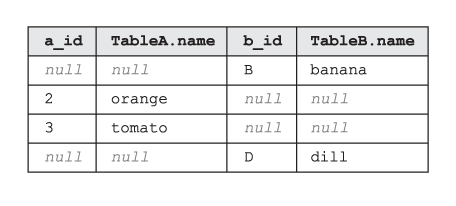A LEFT EXCLUDING JOIN returns all of the records in TableA that don't match any record in TableB.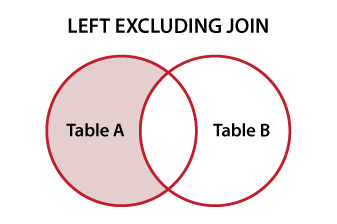```SELECT *
FROM tableA
LEFT JOIN tableB
ON tableA.name = tableB.name
WHERE tableB.name IS NULL
```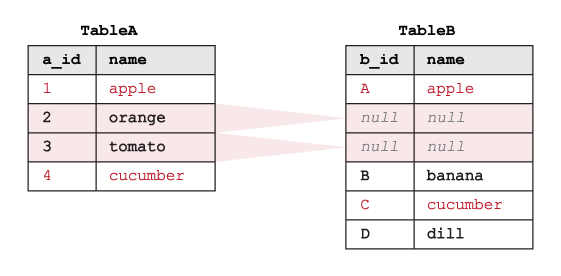The resulting table will be as follows: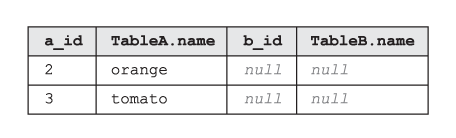A RIGHT EXCLUDING JOIN returns all of the records in TableB that don't match any records in TableA.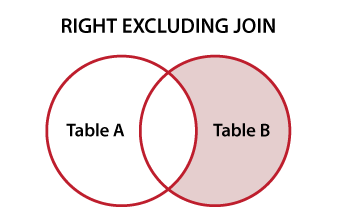```SELECT *
FROM tableA
RIGHT JOIN tableB
ON tableA.name = tableB.name
WHERE tableA.name IS NULL
```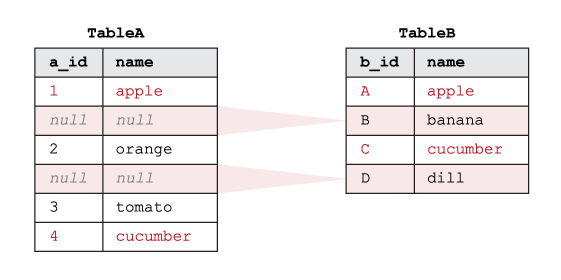The resulting table will be as follows: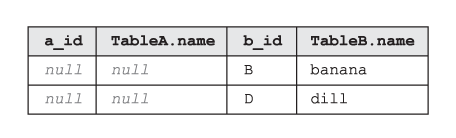### A bunch of useful links: